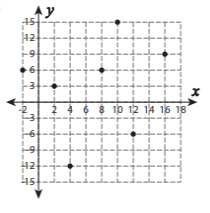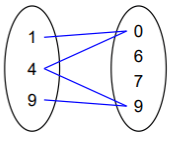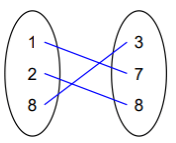# Worksheet on Functions or Mapping | Functions Worksheet with Answers

Those seeking help on Functions or Mapping can make the most out of the Worksheet on Functions or Mapping. Most of the questions prevailing here are based on domain, codomain, and range of a function, identifying functions from Mapping Diagrams, determining whether a set of ordered pairs form a function or not, etc. Attempt all the questions in the Identifying Functions from Mappin Diagrams Worksheets and get a better idea of the concepts within.

For the sake of your convenience, we even listed the step by step solutions to all the sample provided. Solve the  Mapping and Functions Problems on your own and verify with the Answers Provided and test your preparation level.

1. State whether each set of ordered pair represent a function or not?

{(1, 9), (-4, –6), (-6, 5), (3, 8), (7, –16), (–10, 7)}

Solution:

Given Set of Ordered Pairs {(1, 9), (-4, –6), (-6, 5), (3, 8), (7, –16), (–10, 7)}

It is a function since any of the first components aren’t repeated and have unique images in the other set.

2. State whether each set of ordered pair represent a function or not?

{(-4, 3), (5, -7), (10, 5), (9, 6), (6, -3), (5, 8), (1, 3)}

Solution:

Given Set of Ordered Pairs {(-4, 3), (5, -7), (10, 5), (9, 6), (6, -3), (5, 8), (1, 3)}

It is not function since the first components are repeated.

3. State whether the following relation represents a function or not?Solution:

The above arrow diagram represents a function since each element in the set is associated with one element in the other set. Thus it represents a function.

4. A function is given by f(x) = 3x+3. Find the values of

(a) f(1)

(b) f(0)

(c) f(-2)

Solution:

Given f(x) = 3x+3

To find f(1) substitute 1 in place of x in the given function

f(1) = 3*1+3 = 3+3 = 6

To find f(0) substitute 0 in place of x in the given function

f(0) = 3*0+3 = 0+3 = 3

To find f(-2) substitute -2 in place of x in the given function

f(-2) = 3*(-2)+3 = -6+3 = -3

5. Determine whether each set of ordered pairs on the graph represents a function or not?Solution:

Ordered Pairs = {(-2, 6) (2, 3) (4, -12) (8, 6) (10, 15) (12, -6) (16, 9)}

The above graph represents a function since it has first components unique.

6. Determine whether the following Mapping Diagram represents a function or not?Solution:

The above mapping diagram represents a function since each element in the set is mapped with only one element in the other set.

7. Which of the following represents mapping?

a) {(3, 2); (7, 3); (6, 5); (8, 7)}

b) {(4, 8); (5, 12); (4, 14)}

Solution:

a) {(3, 2); (7, 3); (6, 5); (8, 7)}

The Set of Ordered Pairs represent Mapping since all of them have unique images.

b) {(4, 8); (5, 12); (4, 14)}

Set of Ordered Pairs doesn’t represent Mapping Since doesn’t have unique images and the first components are repeated.

8. Determine whether the relationship given in the Mapping Diagram is a function or not?Solution:

Since 4 is mapped with more than one element as output i.e. 0, 9 the relationship given in the above mapping diagram is not a function.

9. Draw Mapping Diagram for the Relation R = {(1, 7) (2, 8) (8, 3)}?

Solution:

Place all the domain values in the left column and write the range value in the right column and draw arrows between them indicating the relationship.